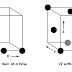## Latest

recent

There are many applications in which the input domain is relatively limited. That is, the number of input parameters is small and the values that each of the parameters may take are clearly bounded. When these numbers are very small (e.g., three input parameters taking on three discrete values each), it is possible to consider every input permutation and exhaustively test processing of the input domain. However, as the number of input values grows and the number of discrete values for each data item increases, exhaustive testing become impractical or impossible.

Orthogonal array testing can be applied to problems in which the input domain is relatively small but too large to accommodate exhaustive testing. The orthogonal array testing method is particularly useful in finding errors associated with region faults—an error category associated with faulty logic within a software component.

To illustrate the difference between orthogonal array testing and more conventional “one input item at a time” approaches, consider a system that has three input items, X, Y, and Z. Each of these input items has three discrete values associated with it. There are 33 = 27 possible test cases. Phadke [PHA97] suggests a geometric view of the possible test cases associated with X, Y, and Z illustrated in figure. Referring to the figure, one input item at a time may be varied in sequence along each input axis. This results in relatively limited coverage of the input domain (represented by the left-hand cube in the figure).

When orthogonal array testing occurs, an L9 orthogonal array of test cases is created. The L9 orthogonal array has a “balancing property .” That is, test cases (represented by black dots in the figure) are “dispersed uniformly throughout the test domain,” as illustrated in the right-hand cube in Figure 17.10. Test coverage across the input domain is more complete.

To illustrate the use of the L9 orthogonal array, consider the send function for a fax application. Four parameters, P1, P2, P3, and P4, are passed to the send function. Each takes on three discrete values. For example, P1 takes on values:

P1 = 1, send it now
P1 = 2, send it one hour later
P1 = 3, send it after midnight

P2, P3, and P4 would also take on values of 1, 2 and 3, signifying other send functions.
If a “one input item at a time” testing strategy were chosen, the following sequence of tests (P1, P2, P3, P4) would be specified: (1, 1, 1, 1), (2, 1, 1, 1), (3, 1, 1, 1), (1, 2, 1, 1), (1, 3, 1, 1), (1, 1, 2, 1), (1, 1, 3, 1), (1, 1, 1, 2), and (1, 1, 1, 3). Phadke [PHA97] assesses these test cases in the following manner:

Such test cases are useful only when one is certain that these test parameters do not interact. They can detect logic faults where a single parameter value makes the software malfunction. These faults are called single mode faults. This method cannot detect logic faults that cause malfunction when two or more parameters simultaneously take certain values; that is, it cannot detect any interactions. Thus its ability to detect faults is limited. Given the relatively small number of input parameters and discrete values, exhaustive testing is possible. The number of tests required is 34 = 81, large, but manageable. All faults associated with data item permutation would be found, but the effort required is relatively high.

The orthogonal array testing approach enables us to provide good test coverage with far fewer test cases than the exhaustive strategy. An L9 orthogonal array for the fax send function is illustrated in figure.

Phadke  assesses the result of tests using the L9 orthogonal array in the following manner:

Detect and isolate all single mode faults.
A single mode fault is a consistent problem with any level of any single parameter. For example, if all test cases of factor P1 = 1 cause an error condition, it is a single mode failure. In this example tests 1, 2 and 3  will show errors. By analyzing the information about which tests show errors, one can identify which parameter values cause the fault. In this example, by noting that tests 1, 2, and 3 cause an error, one can isolate [logical processing associated with “send it now” (P1 = 1)] as the source of the error. Such an isolation of fault is important to fix the fault.

Detect all double mode faults. If there exists a consistent problem when specific levels of two parameters occur together, it is called a double mode fault. Indeed, a double mode fault is an indication of pairwise incompatibility or harmful interactions between two test parameters.

Multimode faults. Orthogonal arrays [of the type shown] can assure the detection of only single and double mode faults. However, many multi-mode faults are also detected by these tests.
Software Engineering-Orthogonal Array TestingReviewed by 1000sourcecodes on 06:38 Rating: 5